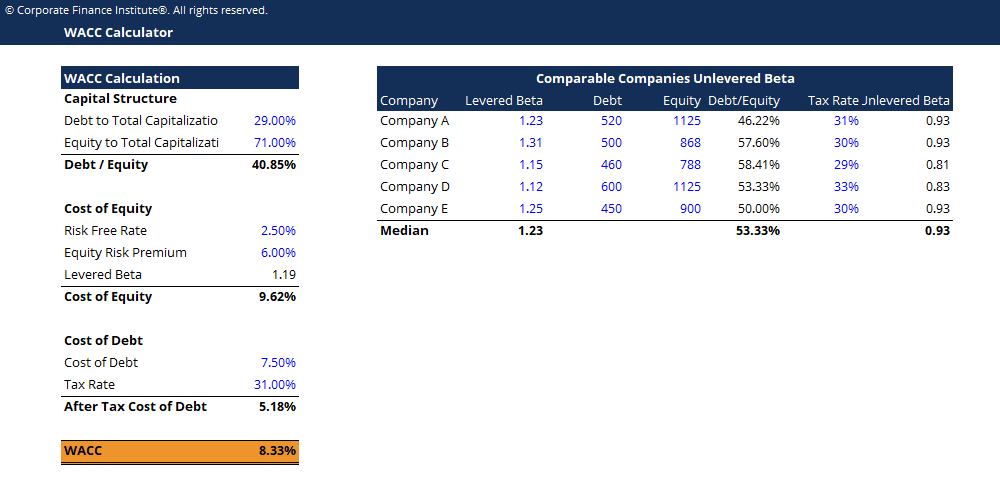# WACC Calculator

## WACC Calculator

This WACC calculator helps you calculate WACC based on capital structure, cost of equity, cost of debt and tax rate.### WACC Calculator

Weighted Average Cost of Capital (WACC) represents a company’s blended cost of capital across all sources, including common shares, preferred shares, and debt.  The cost of each type of capital is weighted by its percentage of total capital and they are added together. The purpose of WACC is to determine the cost of each part of the company’s capital structure based on the proportion of equity, debt, and preferred stock it has.

The WACC formula is: WACC  =  (E/V x Re)  +  ((D/V x Rd)  x  (1 – T))

Where:

E = market value of the firm’s equity (market cap)
D = market value of the firm’s debt
V = total value of capital (equity plus debt)
E/V = percentage of capital that is equity
D/V = percentage of capital that is debt
Re = cost of equity (required rate of return)
Rd = cost of debt (yield to maturity on existing debt)
T = tax rate

### More Free Templates

For more resources, check out CFI’s business templates library to download numerous free Excel modeling, PowerPoint presentation, and Word document templates.

### Financial Analyst Certification

Become a certified Financial Modeling and Valuation Analyst (FMVA)® by completing CFI’s online financial modeling classes and training program!